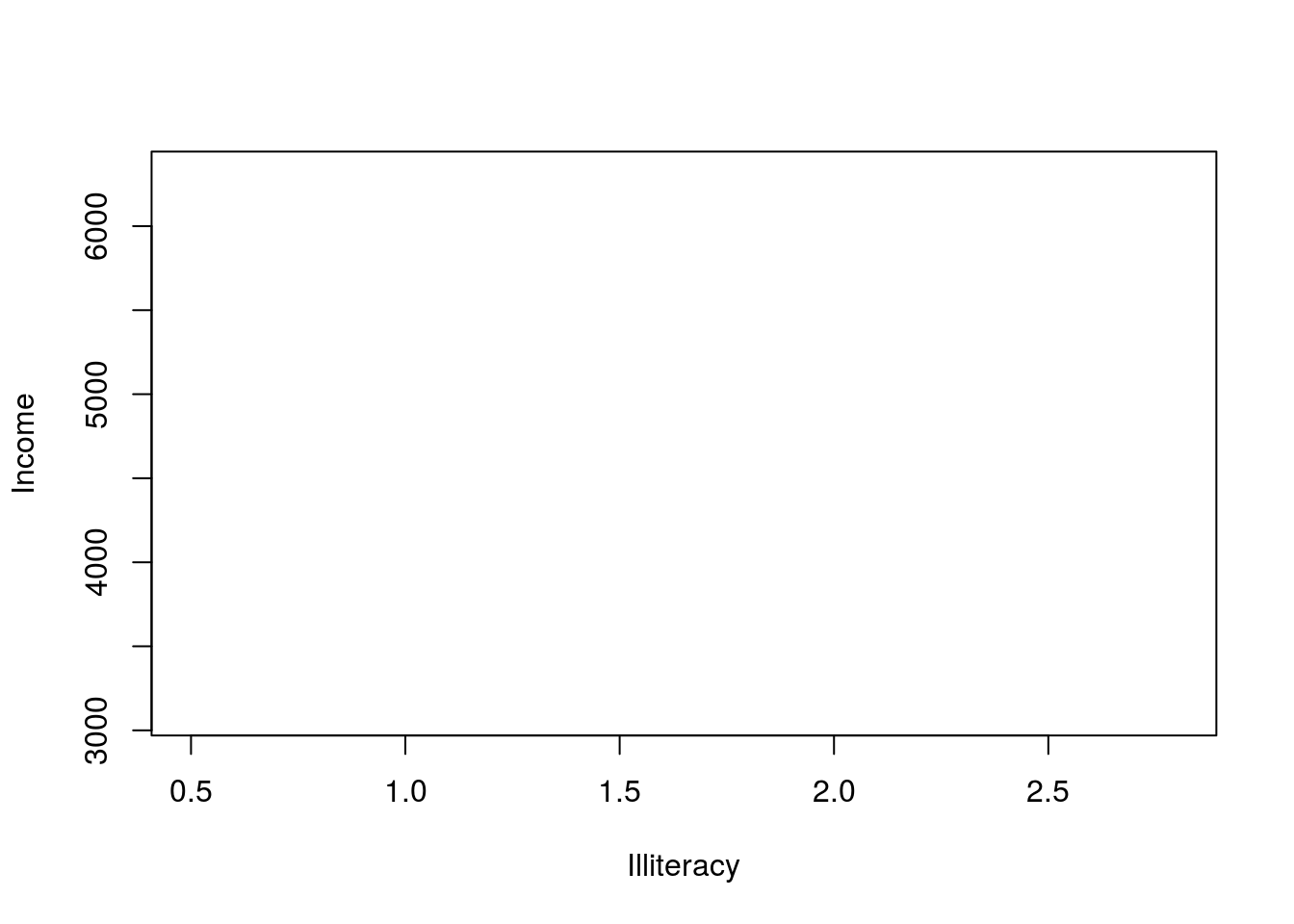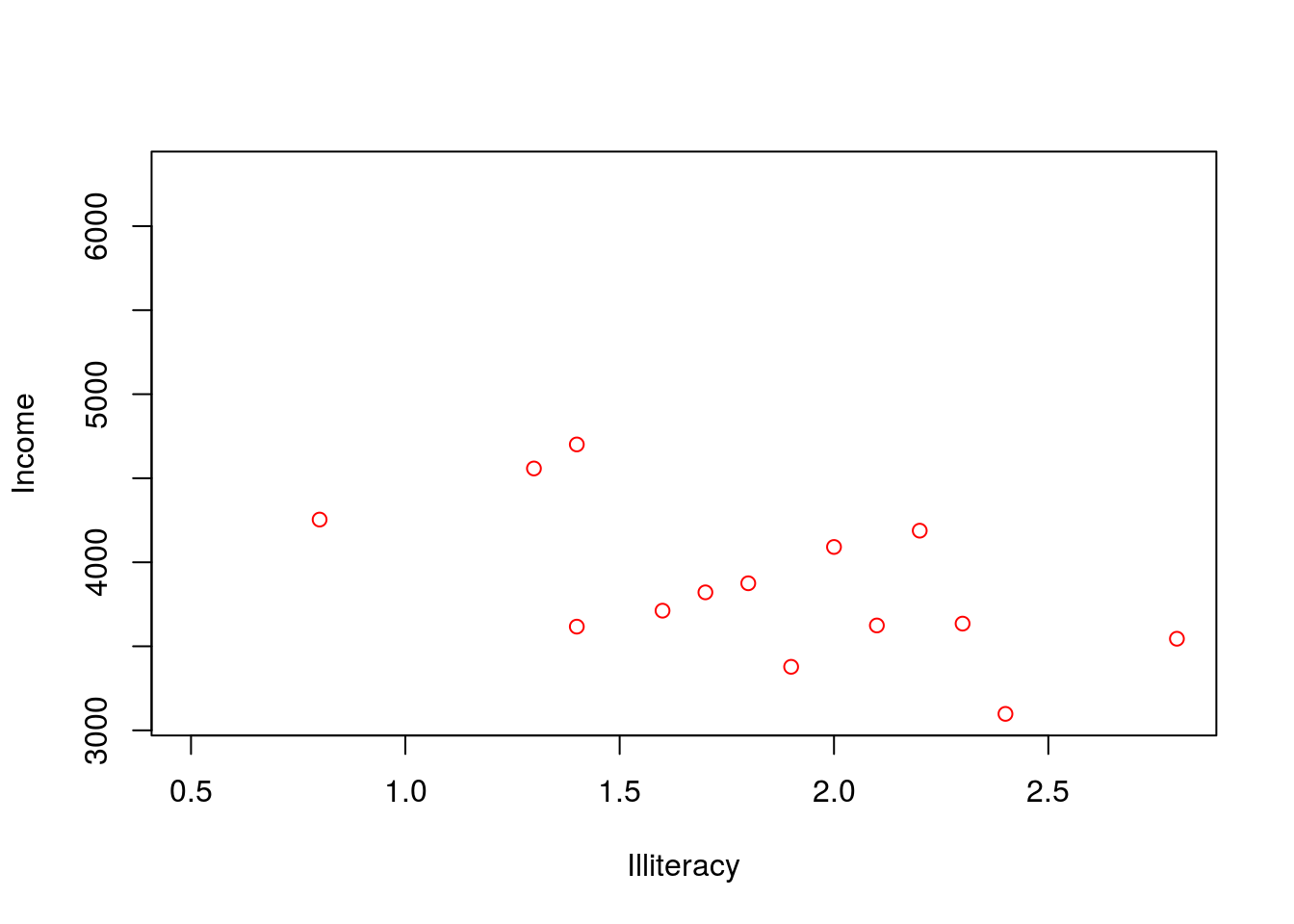Up until now, we have explored how to modify the parameters of graphs themselves, but what if we want to add extra elements or text to the plot?

We can use many functions, including the `points()`, `lines()`, or `text()` functions to do just that. In all three cases, we need to provide some coordinates (x and usually y), and then provide some specific direction in terms of the plotting character (`pch =`), colour (`col =`), line width (`lwd =`) or size (`cex =`) for points; line type (`lty =`) or line width (`lwd =`) for lines; and labels (i.e., the text that goes at each coordinate, as for axis), size, colour, or font for text.

Before we can use these functions, however, we first need to intialize a plot to which these elements can be added.

Using the state dataset we loaded in this lesson for you, plot Income as a function of Illiteracy using the ‘data’ argument, but set the plot type to ‘n’.

``plot(Income ~ Illiteracy, data = state, type = 'n')``We currently have a blank canvas—let’s use the `points()` function to begin filling it in.

The syntax of the `points()` function works largely like the plot function. Go ahead and add points to the plot, specifying Income as a function Illiteracy with the data argument, but subset the data such that we’re only plotting points where HS_grad is less than 50. Make these points ‘red’.

``````plot(Income ~ Illiteracy, data = state, type = 'n')

points(Income ~ Illiteracy, data = state[state\$HS_grad < 50,], col = "red")``````Now do the same thing, but instead add points for HS_grad greater than or equal to 50. Make these points blue.

``````plot(Income ~ Illiteracy, data = state, type = 'n')
points(Income ~ Illiteracy, data = state[state\$HS_grad < 50,], col = "red")

points(Income ~ Illiteracy, data = state[state\$HS_grad >= 50,], col = "blue")``````